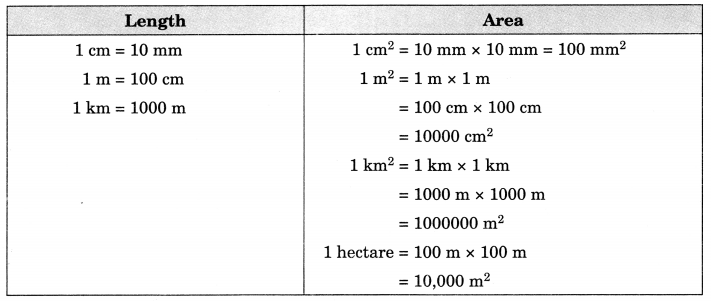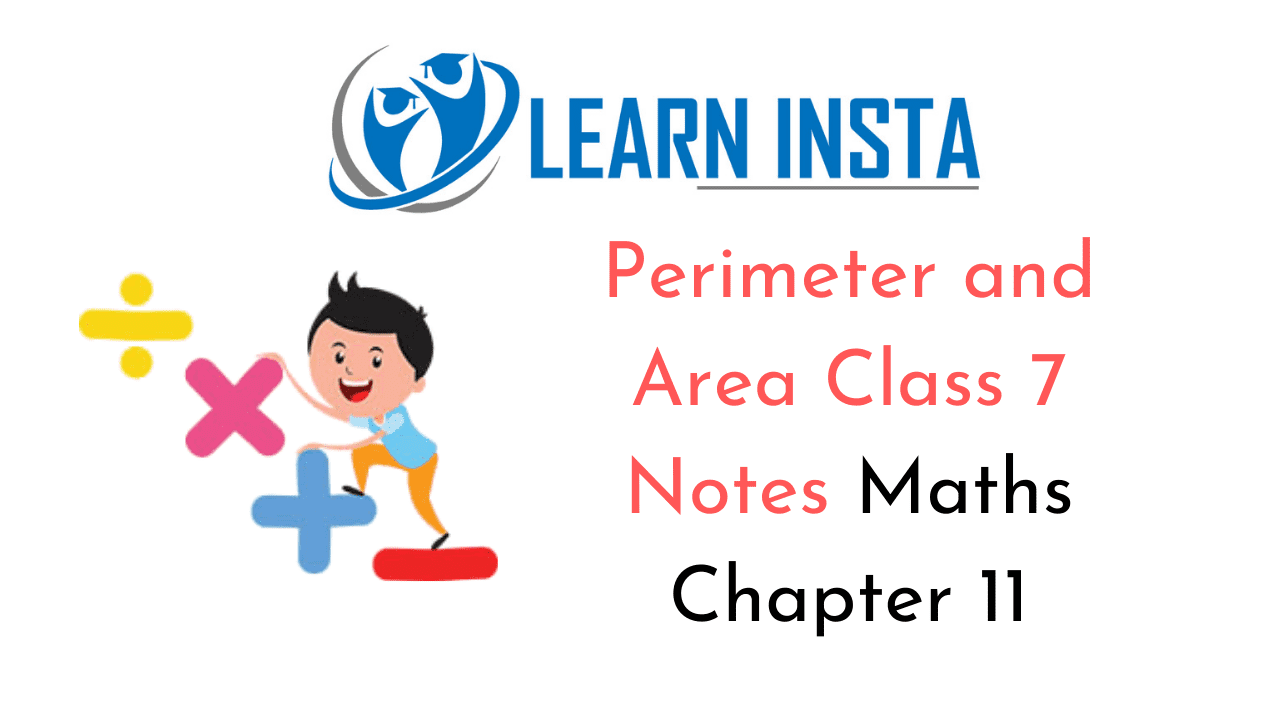On this page, you will find Perimeter and Area Class 7 Notes Maths Chapter 11 Pdf free download. CBSE NCERT Class 7 Maths Notes Chapter 11 Perimeter and Area will seemingly help them to revise the important concepts in less time.

## CBSE Class 7 Maths Chapter 11 Notes Perimeter and Area

### Perimeter and Area Class 7 Notes Conceptual Facts

1. Perimeter is the actual distance around a closed figure.

2. Perimeter of a regular polygon = Number of sides x Length of one side

3. Perimeter of a square = 4 x side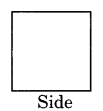4. Perimeter of a triangle = AB + BC + CA (Sum of all sides of triangle)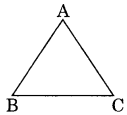5. Perimeter of a rectangle = 2 [length + breadth]
= 2(l+ b)6. Circumference of a circle is the actual distance around it.

7. Ratio of the circumference and the diameter of a circle is a constant

8. The numerical value of π is taken as $$\frac{22}{7}$$ or 3.14. (approximate)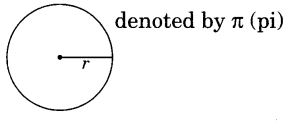9. Circumference of a circle = 2πr, where r is the radius of the circle.

10. Area of a rectangle = length x breadth = l x b11. Area of a triangle = $$\frac{1}{2}$$ x base x height = $$\frac{1}{2}$$ x b x h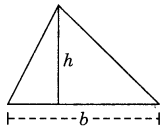12. Area of circle = πr2, where r is the radius of the circle.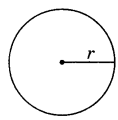13. Area of a parallelogram = base x height14. Area of a square = (Side)2 = l2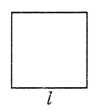Conversion of units